### Home > MC2 > Chapter 7 > Lesson 7.1.5 > Problem7-52

7-52.
1. Use the hundredths grids below to answer the following questions. Homework Help ✎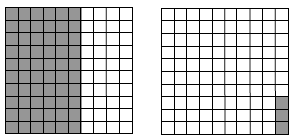1. Give three names for the largest shaded area.

2. Give three names for the smallest shaded area.

3. What are two other names for 120%? Can you show 120% on a single hundreds grid? Explain your thinking.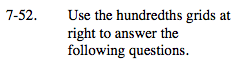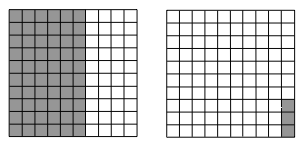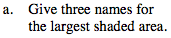Find the number of shaded squares by finding the area represented or by counting each square. If this number is out of 100 total squares, how can you represent this value as a fraction, decimal, or percentage?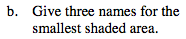See part (a).

$0.03 = 3\%= \frac{3}{100}$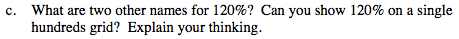How can 120% be represented as a fraction or decimal value?

If there are only 100 squares on a single grid, can you represent 120 squares on this grid?